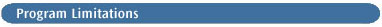#This page outlines the various size limitations that exist within Gaussian 03. These limitations occur in the form of fixed dimension statements and algorithm design limitations, and their overall effect is to limit the size and types of calculation that can be performed.

## Z-matrix Limitations

There are restrictions on the size of a Z-matrix, the maximum number of variables and the maximum number of atoms within a calculation. These are set consistently for a maximum of 20000 real atoms (including ghost but not dummy atoms), and a maximum of 20000 Z-matrix centers (atoms, ghost atoms, and dummy atoms). In addition, the maximum number of variables that can be specified in an optimization is unlimited for Berny optimizations but must not exceed 50 for Murtaugh-Sargent or Opt=EF optimizations (30 for Fletcher-Powell optimizations).

## Basis Set Limitations

Throughout the Gaussian 03 system, basis set limitations manifest themselves in two ways. The main restriction is imposed within the integral evaluation programs and limits the number of primitive gaussian functions and how they are combined into atomic orbital basis functions. Secondly, dimensioning requirements limit the total number of basis functions that can be used in a few of the older of the energy evaluation procedures.

## Integral Program Limitations

To understand fully the limitations in the integral programs, the reader must have some understanding of the concepts presented in discussion of the Gen keyword (input of non-standard bases). In the terminology introduced there, the limitations are as follows: the maximum total number of primitive shells is 60000; the maximum number of primitive d-shells is 20000; the maximum number of primitive f-shells and higher is 20000; the maximum number of contracted shells is 20000. The maximum degree-of-contraction allowed is 100.

The other major restriction that appears in the integral programs is in the manner in which integral labels are packed. These limits apply only when two-electron integrals are written out and can be avoided entirely by using SCF=Direct (which is the default in Gaussian 03). Normally, disk space limitations force the use of direct methods before the following limits are reached.

When the conventional integral storage procedure is selected (in contrast to the Raffenetti ("PK") storage modes ), the suffixes μ, ν, λ, and σ of the two-electron integral (μν|λσ) are packed into a computer word as 8-bit quantities in the UNIX version, and as 16-bit quantities in the UniCOS version. This in effect limits the number of basis functions to 255 under UNIX for conventional calculations in this mode. When the Raffenetti modes are selected (for SCF=Conventional except when Tran=Conventional, Stable=Complex, or CASSCF is also specified), the two linearized suffixes (μν) and (λσ) (where (μν=(μ(μ-1)/2)+ν) are packed into a word. This imposes a theoretical limit of 361 basis functions for conventional calculations on the 32-bit computer systems. These limits do not apply to direct calculations.

## SCF and Post-SCF Limitations

There are only a few other links which have additional dimensioning limits. There is no further restriction for RHF, UHF, ROHF, DFT, MP, CI, QCISD, CC, or BD calculations using the default algorithms. Complex HF calculations are limited to 180 basis functions, and complex MP2 calculations are effectively limited by a requirement of O(N3) words of main memory, and are also limited to f functions. The GVB program is limited to 100 paired orbitals, which is not a restriction in practice.

The remaining restrictions are in some of alternative programs which must be specifically requested. SCF=DM is limited to 255 basis functions, although the preferred SCF=QC can be used with direct SCF and imposes no dimensioning limits. Link 903 (in-core MP2) requires O(N3) words of main memory.

## NBO Dimensions

NBO is dimensioned for 200 atoms and 10000 basis functions.